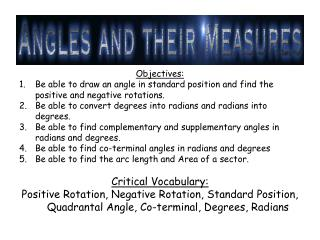DownloadDownload PresentationObjectives:

# Objectives:

Download Presentation## Objectives:

- - - - - - - - - - - - - - - - - - - - - - - - - - - E N D - - - - - - - - - - - - - - - - - - - - - - - - - - -
##### Presentation Transcript

1. Objectives: • Be able to draw an angle in standard position and find the positive and negative rotations. • Be able to convert degrees into radians and radians into degrees. • Be able to find complementary and supplementary angles in radians and degrees. • Be able to find co-terminal angles in radians and degrees • Be able to find the arc length and Area of a sector. Critical Vocabulary: Positive Rotation, Negative Rotation, Standard Position, Quadrantal Angle, Co-terminal, Degrees, Radians

2. Ray: Starts at a point and extends indefinitely in one direction. Angle: Two rays that are drawn with a common vertex Positive Rotation: The angle formed from the initial side to the terminal side rotating ___________________. Negative Rotation: The angle formed from the initial side to the terminal side rotating __________________. Standard Position: ___________________________________. Lies in Quadrant: ____________________________________.

3.  (theta) = the angle measurement. Reference Angle: _________________________________________________________________________________________________________ 1.  = 360 degrees = __________ 2.  = 90 degrees = ___________ 3.  = 180 degrees = ___________ 4.  = 260 degrees = ___________ Example 1:Draw an angle of _______° in standard position  = ________________  = ________________  = ________________ Lies in Quadrant ______ Reference: __________

4. Example 2:Draw an angle of _______° in standard position  = ________________  = ________________  = ________________ Lies in Quadrant ______ Reference: __________ Example 3:Draw an angle of _______° in standard position  = ________________  = ________________  = ________________ Lies in Quadrant ______ Reference: __________

5. Example 4:Draw an angle of _______° in standard position  = ________________  = ________________  = ________________ Lies in Quadrant ______ Reference: __________ Example 5:Draw an angle of _______° in standard position  = ________________  = ________________  = ________________ Lies in Quadrant ______ Reference: __________

6. Page 862-863 #3-9 all, 14 Directions (#3-9): 1. Draw the Angle in Standard Position 2. How many complete rotations 3. What are Alpha and Beta 4. What Quadrant does the Angle Lie in 5. What is the Reference Angle

7. Converting Degrees and radians Example 6: Convert _____ degrees into radians Example 7: Convert _______radians into degrees

8. Complementary Angles: 2 angles sum is 90 degrees __________________ Supplementary Angles: 2 angles sum is 180 degrees _________________ Co-Terminal Angles: Angles in Standard position with terminal sides that coincide Example 8: Give two angles in degrees that are Complementary Example 9: Give two angles in degrees that are Supplementary Example 10: Give two angles in degrees that are Coterminal Example 11: Give two angles in radians that are Complementary Example 12: Give two angles in radians that are Supplementary Example 13: Give two angles in radians that are Coterminal

9. Example 14: Determine two co-terminal angles (one positive and one negative) for the angle 7π/6. Example 15: Find the complement and supplement of the angle π/12

10. Read: Page 861-862 Page 863 #15-37 odds

11. Example 16:Draw an angle of _______° in standard position  = ________________  = ________________  = ________________ Lies in Quadrant ______ Reference: __________ Example 17:Draw an angle of _______° in standard position  = ________________  = ________________  = ________________ Lies in Quadrant ______ Reference: __________

12. Example 18:Draw an angle of _______° in standard position  = ________________  = ________________  = ________________ Lies in Quadrant ______ Reference: __________

13. Worksheet: “Drawing Angles in Standard Position”